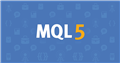# Function templates fail since build 2190

Hello,

hope this is not a duplicate, but i've noticed, the documentation to mql5 concerning function templates is showing code, that does not compile any more, redering the template functionality more or less useless. (And broke my code)

The documentation shows an exmpel at https://www.mql5.com/en/docs/basis/oop/templates:

with the code comparing ( "opeartor <()" ) a templated datatype, which the compiler now errors out as illegal operation in use.

```template<typename T>
T ArrayMax(T &arr[])
{
uint size=ArraySize(arr);
if(size==0) return(0);

T max=arr;
for(uint n=1;n<size;n++)
if(max<arr[n]) max=arr[n];
//---
return(max);
}```

```if(max<arr[n]) // <- Fails to compile: '<' - illegal operation use
```

This concerns up to build 2204.

How to refactor the code to be able to compile this functionality again?

Help would be very appreciated.

Thank you.

Example code:

```// Container class
class ea_dbl
{
// Double storage
public:
double  m_dbl;

// Object handlers
void                    ea_dbl()                                                           { m_dbl = EMPTY_VALUE; }
void                    ea_dbl(const double inVal)                                         { m_dbl = inVal; }
void                    ea_dbl(const ea_dbl& inVal)                                        { m_dbl = inVal.m_dbl; }

// Public interface
public:

// Virtual interface
virtual double                  Get()                                                        const { return(GetRawDouble()); }

// Default interface
virtual double                  GetRawDouble()                                               const { return(m_dbl); }
virtual double                  GetAbs()                                                     const { return(fabs(m_dbl)); }

virtual bool                    GetEmpty()                                                   const { return(m_dbl == EMPTY_VALUE); }
virtual void                    SetEmpty()                                                         { m_dbl = EMPTY_VALUE; }

// Operators
public:

// Generic Operators: Operators: !, ++, --

// ea_dbl selfreferencing operators
virtual bool                    operator !  ()                                               const { return( (m_dbl == EMPTY_VALUE) || (m_dbl <= MathPow(10, ((Digits()+3) * -1))) ); }
virtual void                    operator ++ ()                                                     { m_dbl += MathPow(10, (Digits() * -1)); }
virtual void                    operator -- ()                                                     { m_dbl -= MathPow(10, (Digits() * -1)); }

// Operators: +, -, *, /, %, =, +=, -=, *=, /=, %=

// double as input
virtual double                  operator +  (const double inVal)                             const { return(m_dbl + inVal); }
virtual double                  operator -  (const double inVal)                             const { return(m_dbl - inVal); }
virtual double                  operator *  (const double inVal)                             const { return(m_dbl * inVal); }
virtual double                  operator /  (const double inVal)                             const { return(m_dbl / inVal); }

virtual void                    operator =  (const double inVal)                                   { m_dbl =  inVal; }
virtual void                    operator += (const double inVal)                                   { m_dbl += inVal; }
virtual void                    operator -= (const double inVal)                                   { m_dbl -= inVal; }
virtual void                    operator *= (const double inVal)                                   { m_dbl *= inVal; }
virtual void                    operator /= (const double inVal)                                   { m_dbl /= inVal; }
};

template<typename T> T ArrayMax(T &arr[])
{
uint size = ArraySize(arr);
if(size == 0) return(NULL);

T max = arr;
for(uint n = 1; n < size; n++)
{ if(max < arr[n]) max = arr[n]; }

return(max);
}

int OnInit()
{
ea_dbl test[];
ArrayResize(test, 10);

ea_dbl result = ArrayMax<ea_dbl>(test);

return(INIT_SUCCEEDED);
}

//+------------------------------------------------------------------+
//| Expert deinitialization function                                 |
//+------------------------------------------------------------------+
void OnDeinit(const int reason)
{

return;
}
```Documentation on MQL5: Language Basics / Object-Oriented Programming / Function templates
• www.mql5.com
Overloaded functions are commonly used to perform similar operations on various data types. ArraySize() is a simple example of such function in MQL5. It returns size of any type of array. In fact, this system function is overloaded and the entire implementation of such an overload is hidden from MQL5 application developers: It means that MQL5...

Dominik Egert:

Hello,

hope this is not a duplicate, but i've noticed, the documentation to mql5 concerning function templates is showing code, that does not compile any more, redering the template functionality more or less useless. (And broke my code)

The documentation shows an exmpel at https://www.mql5.com/en/docs/basis/oop/templates:

with the code comparing ( "opeartor <()" ) a templated datatype, which the compiler now errors out as illegal operation in use.

This concerns up to build 2204.

How to refactor the code to be able to compile this functionality again?

Help would be very appreciated.

Thank you.

Example code:

I am very sorry for bothering. - My mistake.

To solve the issue above, the Object in use needs to have a comparison operator in place, which was missing.

i added following lines to the object ea_dbl, which enables the compiler to successfully generate comparison-code to the object, which then leads to working code again.

```        virtual bool                    operator == (const ea_dbl &inVal)                             const { return(m_dbl == inVal.m_dbl); }
virtual bool                    operator != (const ea_dbl &inVal)                             const { return(!operator == (inVal)); }
virtual bool                    operator >  (const ea_dbl &inVal)                             const { return(m_dbl >  inVal.m_dbl); }
virtual bool                    operator <  (const ea_dbl &inVal)                             const { return(m_dbl <  inVal.m_dbl); }
virtual bool                    operator >= (const ea_dbl &inVal)                             const { return(m_dbl >= inVal.m_dbl); }
virtual bool                    operator <= (const ea_dbl &inVal)                             const { return(m_dbl <= inVal.m_dbl); }
```

Thank you everybody, maybe this helps someone else, when working with templates and objects.

Always implement the basic operators to an object.

Greetings.

Normally you only need to define == and < for your classes. The others, are just defined generically in terms of the first two.
```template <typename T>
bool     operator>(const T& lhs, const T& rhs){    return   rhs < lhs;         }
template <typename T>
bool     operator<=(const T& lhs, const T& rhs){   return !(rhs < lhs);        }
template <typename T>
bool     operator>=(const T& lhs, const T& rhs){   return !(lhs < rhs);        }
template <typename T>
bool     operator!=(const T& lhs, const T& rhs){   return !(lhs == rhs);       }```
The above didn't compile on build 840. Might now.
Reason: